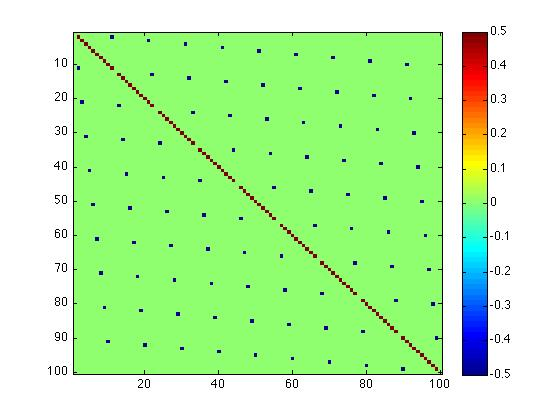# Random subspaces of a tensor product (II)

In this short post, I would like to discuss a special case of the construction introduced in the first part of the series, that is compute the set $K_{A_n}$, where $A_n \subset \mathbb C^n \otimes \mathbb C^n$ is the anti-symmetric subspace of the tensor product. This example plays an important role in the additivity problem for the minimum output entropy of quantum channels, as it was shown in [ghp].

1. Anti-symmetric vectors and matrices

For order two tensors $x \in \mathbb C^n \otimes \mathbb C^n$, there are only two symmetry classes, the symmetric and the antisymmetric vectors. The antisymmetric vectors form a vector space
$!A_n = \mathrm{span}\left \{ \frac{1}{\sqrt 2} (e_i \otimes e_j – e_j \otimes e_i) \, : \, 1 \leq i < j \leq n \right \}$ where the vectors in the span can be shown to form an orthonormal basis of the $\binom n 2$-dimensional subspace $A_n$, whenever $e_i$ form a basis of $\mathbb C^n$. Let $P_n \in \mathcal M_{n^2}(\mathbb C)$ be the orthogonal projection on the subspace $A_n$. It is easy to see that $P_n$ has entries in ${0,\pm 1/2}$ and it looks as below ($n=10$).Via the usual isomorphism $\mathbb C^n \otimes \mathbb C^n \simeq \mathbb C^n \otimes (\mathbb C^n)^* = \mathcal M_n(\mathbb C)$, one can see antisymmetric tensors as antisymmetric (or skew-symmetric) matrices: one simply has to rearrange the $n^2$ complex coordinates of the tensor in an $n \times n$ matrix, respecting the ordering of bases. Note that in this way we obtain antisymmetric ($X^t = -X$) and not anti-Hermitian ($X^* = -X$) elements.

2. Singular values of vectors in the antisymmetric subspace

It is well known that antisymmetric (complex) matrices can be 2-block diagonalized using orthogonal rotations
$!X = O \begin{bmatrix} 0 & \lambda_1 & & & & \\ -\lambda_1 & 0 & & & & \\ & & 0 & \lambda_2 & & \\ & & -\lambda_2 & 0 & & \\ & & & & \ddots & \\ & & & & & 0 \end{bmatrix}O^t.$

The matrix in the middle has either diagonal blocks of size 2, as shown, or null diagonal elements. Hence, the non-zero eigenvalues of an antisymmetric matrix come in pairs $\{ \pm \lambda_i \}$. Since antisymmetric matrices are normal, their singular values are just the moduli of the eigenvalues, so non-zero singular values have multiplicity at least 2. We conclude that
$!K_{A_n} \subset \left\{ (\lambda_1, \lambda_1, \lambda_2, \lambda_2, \ldots) \, : \, \lambda_i \geq 0, \, \sum_{i=1}^{\lfloor n/2 \rfloor} \lambda_i = 1/2\right \}.$

Actually, it is easy to see we have equality, since the vector
$! x_\lambda = \sum_{i=1}^{\lfloor n/2 \rfloor} \sqrt{ \lambda_i } (e_i \otimes e_{\lfloor n/2 \rfloor+i} – e_{\lfloor n/2 \rfloor+i} \otimes e_i)$
is a unit norm element of $A_n$. We summarize everything in the following theorem, where $\prec$ denotes the majorization relation.

Theorem 1. The set of all possible singular values of antisymmetric vectors inside $\mathbb C^n \otimes \mathbb C^n$ is given by
$!K_{A_n} = \{ (\lambda_1, \lambda_1, \lambda_2, \lambda_2, \ldots) \in \Delta_n \, : \, \lambda_i \geq 0, \, \sum_{i=1}^{\lfloor n/2 \rfloor} \lambda_i = 1/2 \}.$
In particular, the set $K_{A_n}$ is convex and we have that $\lambda \prec (1/2, 1/2, 0, \ldots, 0)$ for all $\lambda \in K_{A_n}$. Hence, the minimum entropy of a vector inside $K_{A_n}$ is 1 bit.

References

[ghp] A. Grudka, M. Horodecki and L. Pankowski. Constructive counterexamples to the additivity of the minimum output Rényi entropy of quantum channels for all $p>2$. J. Phys. A: Math. Theor. 43 425304.